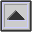SOUND TRANSMISSION LOSS or TRANSMISSION LOSS (TL)

A measure of the reduction in magnitude of SOUND INTENSITY in a TRANSMISSION system.

The loss due to geometric spreading (see SOUND PROPAGATION) is called divergence loss. By implication, transmission loss is also a measure of SOUND INSULATION in a structure. It is calculated from the equation:

TL = 10 log10 (1/s) (dB)

where s is the SOUND TRANSMISSION COEFFICIENT.

home